# 条件概率

## 独立事弹子 玻璃球件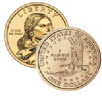## 相关事件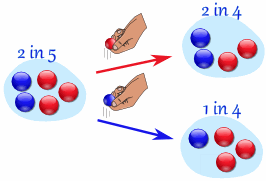### 例子：布袋里的弹子

• 如果第一次拿的是的，第二次拿到蓝弹子的可能性是四分之二
• 如果第一次拿到是的，第二次拿到蓝弹子的可能性是四分之一

"放回原位"

• 放回：事件是独立的（可能性不变）
• 放回：事件是相关的（可能性改变）

## 树图## 记法

P(A) 的意思是 "事件 A 的概率"

P(A) = 2/5

• 如果第一次拿到的是蓝色，第二次拿蓝色的可能性是 1/4
• 如果第一次拿到的是红色，第二次拿蓝色的可能性是 2/4

P(B|A) 的意思是 "在事件 A 发生的条件下，事件 B 发生的概率"

P(B|A) 也叫在 A 发生的情况下 B 发生的 "条件概率"。

P(B|A) = 1/4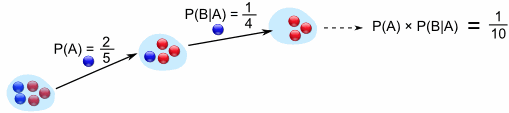"事件 A 与 事件 B 的概率等于

### 例子：在一副扑克牌里拿 2张国王

P(A) = 4/52

P(B|A) = 3/51

P(A 与 B) = P(A) × P(B|A) = (4/52) × (3/51) = 12/2652 = 1/221

## 寻找隐藏数据

 开始： P(A 与 B) = P(A) × P(B|A) 换边： P(A) × P(B|A) = P(A 与 B) 除以 P(A)： P(B|A) = P(A 与 B) / P(A)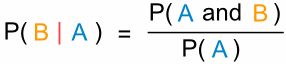"在事件 A 发生的条件下事件 B 发生的概率等于

### 例子：冰淇淋

P(草莓|巧克力) = P(巧克力 与 草莓) / P(巧克力)

0.35 / 0.7 = 50%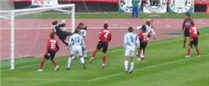## 复杂例子：足球赛

• 如果是小山做教练，你当守门员的可能性是 0.5
• 如果是阿力做教练，你当守门员的可能性是 0.3P(A 与 B) = P(A) x P(B|A)（小山做教练的 0.6 的概率乘以小山让你当守门员的 0.5 的概率等于 0.3 的概率。）（42% 的可能性）

### 检验0.3 + 0.3 + 0.12 + 0.28 = 1

## 朋友和随机数

4个朋友（小李、蛮牛、小王 和 大山），每人从1到5之间随机选一个数。他们其中任何两个人选中相同数字的可能性是多少？

### 首先，小李和蛮牛选同一个数的可能性是多少？(1/5 + 4/5 = 5/5 = 1)

### 现在把小王也算进去……

• 如果小李和蛮牛的数相同的，小王只有一个数来和他的数比较。
• 但如果小李和蛮牛的数不是相同的，小王便有两个数来比较。(4/5) × (2/5) = 8/25

(4/5) × (3/5) = 12/25

(5/25) + (8/25) + (12/25) = 25/25 = 1

### 如果我们把大山也算进去呢？(4/5) × (3/5) × (2/5) = 24/125

1 - (24/125) = 101/125

（连树图也不需要！）

（用 1 减去"否"的概率就是"是"的概率）

（我们在 同一个生日 这网页里有这个技巧的具体说明。）